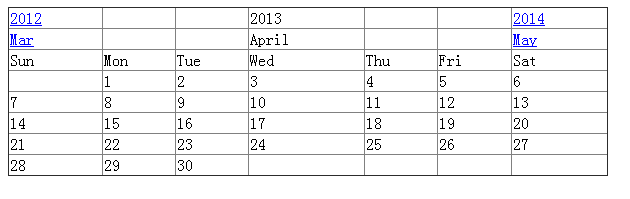# PHP中的日期和时间

• mktime():int mktime(int \$hour, int \$minute, int \$second, int \$month, int \$day, int \$year)，返回unix时间戳。对于传入的参数，可以从右往左依次省略，如果省略的话，默认的是当前时间的某个值，比如省略了\$year 则默认是 2013。

• date():string date(string format, int timestamp)，返回将整数 timestamp 用 format 格式化后的时间格式，timestamp 可以省略，若省略则默认是当前的时间戳(time())。

• strtotime():这个函数的功能很强大，通过例子我们就能理解了。

• microtime():返回当前时间的 unix 时间戳和微秒数。

```COPYPHP```//date_default_timezone_set("Asia/Shanghai");
echo 'date_default_timezone:'.date_default_timezone_get().'<br/>';

echo 'time():'.time().'<br/>';

echo 'date("Y/m/d H:i:s"):'.date('Y/m/d H:i:s').'<br/>';
echo 'date("Y/m/d H:i:s", 1365665148)'.date("Y/m/d H:i:s", 1365665148).'<br/>';

echo 'microtime():'.microtime().'<br/>';
echo 'microtime(true):'.microtime(true).'<br/>';

echo 'mktime(14, 43, 0, 4, 11, 2013):'.mktime(14, 43, 0, 4, 11, 2013).'<br/>';
echo 'mktime(14, 43, 0, 4):'.mktime(14, 43, 0, 4).'<br/>';

echo 'strtotime("April 11th 2013 14:43:00"):'.strtotime('April 11th 2013 14:43:00').'<br/>';
echo 'strtotime("April"):'.strtotime('April').'<br/>';
echo 'strtotime("+1 day"):'.date("Y/m/d l H:i:s", strtotime('+1 day')).'<br/>';
echo 'strtotime("-1 month"):'.date("Y/m/d l H:i:s", strtotime('-1 month')).'<br/>';
echo 'strtotime("-2 year"):'.date("Y/m/d l H:i:s", strtotime('-2 year')).'<br/>';
echo 'strtotime("next friday"):'.date("Y/m/d l H:i:s", strtotime('next friday')).'<br/>';
echo 'strtotime("last saturday"):'.date("Y/m/d l H:i:s", strtotime('last saturday')).'<br/>';``````1. 一个月的第一天（1 号）的时间戳，这个时间戳的作用是获取该月的上一个月、下一个月和上一年、下一年的时间节点；

2. 为了展示一个月的完整的日历，我们需要：1 号距离星期日前面有几个空白天（假如星期日是一个月的第一天），该月一共有多少天，该月最后剩余的空白。如果你还想弄一个回到今天的链接，那么我还需要获取今天的日期；

3. 我们链接的跳转可以设置为向 url 传递参数，然后页面根据获取的参数展示相应的月历；

4. 上一年的链接制作：上一年的年份和现在的月份；下一年的链接制作：下一年的年份和现在的月份；

5. 上一个月的链接制作：上一个月所在的年份和上一个月的月份；下一个月的链接制作：下一个月所在的年份和下一个月的月份。为什么不能直接用现在的年份呢？你懂得。

6. 按照格式填入到表格中，然后输出。

7. 完成。

```COPYPHP```\$g = \$_REQUEST;
\$year  = isset(\$g['year'])?\$g['year']:date("Y");    //获取\$year
\$month = isset(\$g['month'])?\$g['month']:date("F");    //获取\$month
\$start_day  = strtotime("{\$month} 1 {\$year}");        //\$year \$month的第一天的时间戳
\$day_num    = date('t', \$start_day);                //一个月的天数
\$date_range = range(1, \$day_num);                    //
\$pre_month  = strtotime("-1 month", \$start_day);    //上一月的第一天的时间戳
\$next_month = strtotime("+1 month", \$start_day);    //下一个月的第一天的时间戳
\$pre_year   = strtotime("-1 year", \$start_day);        //
\$next_year  = strtotime("+1 year", \$start_day);``````

range() 函数创建并返回一个包含指定范围的元素的数组。

```COPYPHP```define(EMP, ' ');
\$html = "<a href='{\$_SERVER['SCRIPT_NAME']}?year=%s&month=%s'>%s</a>";
\$calender_data = array();
\$calender_data[] = sprintf(\$html, date('Y', \$pre_month), \$month, date('Y', \$pre_year));
\$calender_data[] = EMP;
\$calender_data[] = EMP;
\$calender_data[] = \$year;
\$calender_data[] = EMP;
\$calender_data[] = EMP;
\$calender_data[] = sprintf(\$html, date('Y', \$next_year), \$month, date('Y', \$next_year));

\$calender_data[] = sprintf(\$html, date('Y', \$pre_month), date('F', \$pre_month), date('M', \$pre_month));
\$calender_data[] = EMP;
\$calender_data[] = EMP;
\$calender_data[] = \$month;
\$calender_data[] = EMP;
\$calender_data[] = EMP;
\$calender_data[] = sprintf(\$html, date('Y', \$next_month), date('F', \$next_month), date('M', \$next_month));``````

```COPYPHP```\$calender_data[] = 'Sun';
\$calender_data[] = 'Mon';
\$calender_data[] = 'Tue';
\$calender_data[] = 'Wed';
\$calender_data[] = 'Thu';
\$calender_data[] = 'Fri';
\$calender_data[] = 'Sat';``````

```COPYPHP```\$start_blank = date('N', \$start_day)%7;
for(\$i=0; \$i<\$start_blank; \$i++){
\$calender_data[] = EMP;
}
foreach(\$date_range as \$v){
\$calender_data[] = \$v;
}
\$end_blank = (7-(\$day_num+\$start_blank)%7)%7;
for(\$i=0; \$i<\$end_blank; \$i++){
\$calender_data[] = EMP;
}``````

```COPYPHP```\$h = '<table border="1px" cellspacing="0px" style="border-collapse:collapse; width:600px; margin-top:10px;"><tr>';
for(\$i=0, \$t=count(\$calender_data); \$i<\$t; \$i++){
\$h .= "<td>{\$calender_data[\$i]}</td>";
if((\$i+1)%7==0){
\$h .= "</tr></tr>";
}
}
\$h .= '</table>';
echo \$h;``````### 公众号：### 公众号：